## Introduction

The “double tap” is a gesture that today is a crucial part of many of our daily actions.

So, the first step towards this goal is to make sure we have all the materials to develop and test the algorithm. We will use the X-NUCLEO-IKS01A2 board, that is easy to use and install on PYNQ.

The next step is to create an appropriate design for communication with the X-NUCLEO-IKS01A2; in fact you can access to the accelerometer on this board through a simple I2C communication.

Below is the Vivado design that we will use.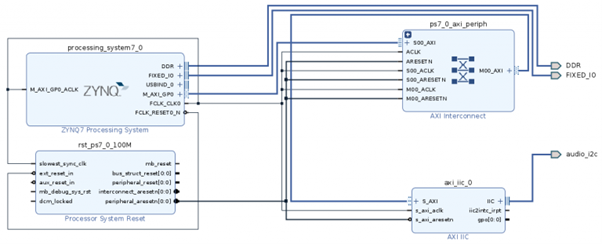For all the details on how to get to this design and how to manage the various constraints, we recommend the following article: spi-i2c-uart-on-pynq-a-pl-approach.

## First Test

To verify our setup, you can use a Jupyter Notebook with those classes: LSM6DSL (contained in LSM6DSL.py) and IIC (contained in LSM6DSL.py with some modifications).

So let’s start coding:

``````
from pynq import Overlay
from time import sleep, time
import pynq.lib as lib
from utils.classes import LSM6DSL

import matplotlib.pyplot as plt
%matplotlib inline

ol = Overlay("./design_oid_ble_iic/design_1.bit")

``````

To get started, you need to import the libraries and instantiate the Overlay object.

``````
class IIC:

overlay = None
i2c = None
_lsm6dsl = None

def __init__(self, overlay_in):
self.overlay = overlay_in
self.i2c = lib.AxiIIC(self.overlay.ip_dict['axi_iic_0'])

def init_LSM6DSL(self):
self._lsm6dsl = LSM6DSL(self.i2c)

gyroscopeX = self._lsm6dsl.getGyroscopeXAxis()
GAxis = self._lsm6dsl.getGAxes()
return gyroscopeX, GAxis
IIC_obj = IIC(ol)

``````

Above is the class IIC with the modifications to be able to read the values from the accelerometer.

``````
acc_vec_x = []
acc_vec_y = []
acc_vec_z = []

gyr_vec_z = []
gyr_vec_y = []
gyr_vec_x = []

X_axis = []
count = 0
IIC_obj.init_LSM6DSL()
while True:
try:

print('Acc[mg]:     [{}, {}, {}]'.format(round(accel1,2), round(accel1,2), round(accel1,2)))
print('Gyr[mdps]:   [{}, {}, {}]'.format(round(Gaxis,2),  round(Gaxis,2),  round(Gaxis,2)))
print('')
acc_vec_x.append(round(accel1,2))
acc_vec_y.append(round(accel1,2))
acc_vec_z.append(round(accel1,2))

X_axis.append(count)
count = count + 1
sleep(0.1)
except Exception as e:
print("error: {}".format(e))
sleep(2)

The code above contains the main while loop in which the acceleration values perceived on each of the three axes are read from the sensor
plt.plot(X_axis, acc_vec_x, label= "X")
plt.plot(X_axis, acc_vec_y, label= "Y")
plt.plot(X_axis, acc_vec_z, label= "Z")
plt.title()
plt.show()

``````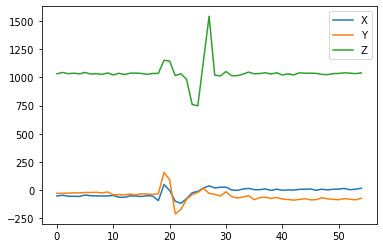For a better visualization and understanding of the values, we have shown the data received from the accelerometer in a graph.The sensor is very sensitive to smallest fluctuations.

In the previous image, a simple movement describes a double tap, and we made this two simple deductions:

1. the position of the sensor, in its initial state, is “vertical”, because no force is perceived on the X and Y axes;
2. the force impressed is mainly along the Z axis.

## LSM6DSL: why and what it is

The X-NUCLEO-IKS01A2 board demonstrates how the obtained data are sufficiently precise for our purpose. Unfortunately, since this board is directly linked to the Pynq-Z2, it made difficult to carry out the tests and development of the algorithm.

For this reason we have decided to use only the accelerometer sensor, therefore to use the LSM6DSL.

Despite its small size, The LSM6DSL is a system-in-package featuring a high-performance 3-axis digital accelerometer and 3-axis digital gyroscope.

Furthermore, as explained in the datasheet, this module is perfect for implementing algorithms such as double tap, motion detection, tilt, pedometer function and many others.

At this point we need to verify that it is compatible with the Pynq-z2.

This module supports two communication protocols:

• SPI, in the following connections, CS, SPC, SDI and SDO;
• I2C in the SDA and SCL configuration.

With the possibility to connect to the LSM6DSL module via I2C protocol, we can reuse the previously used IIC design and class.

Furthermore, in order to interface with the accelerometer, it is possible to use the same functions contained in the LSM6DSL.py file.

Perfect!! Now we can move on to the next step and then to the Double-Tap algorithm!

## Double-Tap Algorithm

Now the only thing missing is the algorithm.

The idea behind the algorithm comes out after some experiments with the new sensor, in which we tried to reproduce a possible action that mimics the double tap gesture.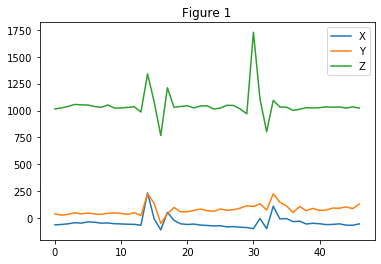After a few attempts, we have recorded the values of the accelerometer in this image.

From the image above we can understand that the preferred position is the vertical one, so we can avoid the X and Y values.

Now we can see that the movement impressed on the sensor produces what appear to be two very distinct oscillations.

The first is our “target signal”, i.e. double tapping in the form of accelerations.

We can describe it by three rapid and consecutive variations of the values:

1. a first rapid growth of values, “The first touch”;
2. then, an equally rapid decrease, the “shock” between one touch and the next;
3. finally a second growth in values, which indicates “The second touch”.

The second swing in the figure was produced with the intention of performing a double tap, but as we can see the second peak, the one that identifies the second tap, does not seem to be intense enough.

Considering now that there can be different intensities with which this gesture can be imprinted, the first point was to create Thresholds.

## Threshold

There are three thresholds though, one for each peak just explained. This allowed us to manage the sensitivity of the various touches separately.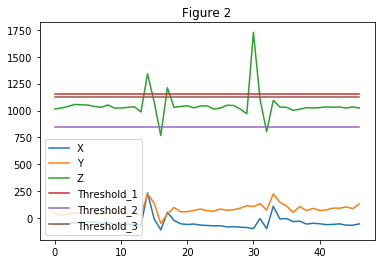For example, by setting the thresholds as in figure 2, only the first oscillation is recognized as a double tap, while the second will be discarded precisely for the second peak which is not sufficiently intense.But if instead we want to allow the second oscillation to be considered as a double tap, then we could intervene on the third threshold by lowering its value.

While this is correct reasoning, thresholds alone are not enough.

There are countless wrong examples but they can be recognized as double taps if only thresholds are used.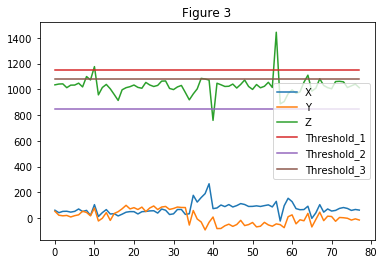In the case of Figure 3, a possible code, which makes use exclusively of these thresholds, you will recognize a double tap even if it does not exist.

We leave it to the reader to find where this fake is located.

From this problem we introduce the Time Window, to exclude the noise from the recognition.

## Time Windows

The Time Windows are introduced to set a time limit, within which the gesture must be performed.

So, the initial idea was to create a unique Time Window, which begins at the first perceived shock. This fact allows you to recognize only those variations determined by the Time Window.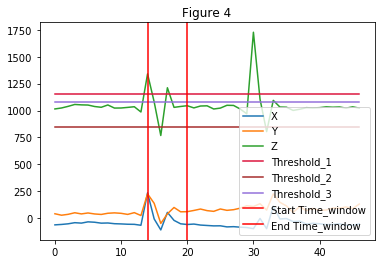We can extend this method can finally by integrating the concept of “Quiet State”. This consists of a time interval in which, in order to recognize the double tap, you don’t need to record values that exceed the thresholds. You can create a more flexible system using more thresholds.

In Figure 5 there is an example with a Time Window and a Quiet State that helps the correct the gesture recognition.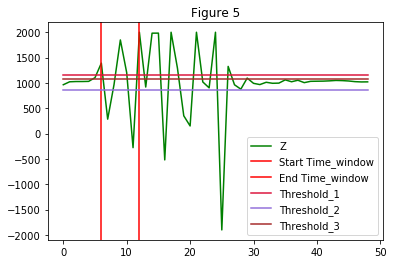A further step consists in breaking down the Time Window into sub-components, in order to individually manage the times in which the various peaks and the Quiet State should occur.

Thus, we create three subcomponents of the Time Window:

1. one for the second peak
2. then, one for the third peak
3. finally, one for the Quiet State

The code of the function that recognizes the Double-Tap gesture is below

## Conclusion

In this article we have seen how to implement a double-tap on PYNQ using an accelerometer. For the future articles, we will see how can use it in a specific application and the main features.

Giacomo Di Maggio, MakarenaLabs